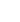10min:
MUTUAL CO-ASSIGNMENT OF THE CALCULATED VIBRATIONAL FREQUENCIES IN THE GROUND AND LOWEST EXCITED ELECTRONIC STATES.

YURII N. PANCHENKO, Laboratory of Molecular Spectroscopy, Division of Physical Chemistry, Department of Chemistry, Lomonosov Moscow State University, Moscow 119991, Russian Federation..

The shifts of the molecular vibrational frequencies when going from the ground electronic state to the lowest excited electronic states pose some problems for the mutual co-assignment of the calculated vibrational frequencies in the different excited states. The trans -\chemC2 O2 F2 shift of the frequency of the symmetrical(C=O) stretching vibration between the S0 and T1 is 373~cm-1.

The feasibility of mutual co-assignments of the vibrational frequencies in these electronic states has been demonstrated for trans -\chemC2 O2 F2. Matrices analogous to the Duschinsky matrix were used to juxtapose the ag vibrational frequencies of this molecule calculated at the CASPT2/cc-pVTZ level in the ground S0 and excited triplet T1 and singlet S1 electronic states. The analog of the Duschinsky matrix D was obtained for this molecule using the equation D = (LI)-1 LII where LI and LII are the matrices of the vibrational modes (normalized atomic displacements) obtained by solving the vibrational problems for the S0 and T1 electronic states, respectively. Choosing the dominant elements in columns of the D matrix and permuting these columns to arrange these elements along the diagonal of the transformed matrix D^makes it possible to establish the correct mutual co-assignments of the calculated ag vibrational frequencies of the trans -\chemC2 O2 F2 molecule in the S0 and T1 electronic states. The analogous procedure was performed for the trans -\chemC2 O2 F2 molecule in the T1 and S1 excited electronic states. The recent reassignments of the2 and3 calculated vibrational frequencies in the trans -\chemC2 O2 F2 molecule in the ground state were also obtained for the triplet T1 and singlet S1 excited electronic states.

The approach set forth in this text makes it possible to juxtapose the calculated vibrational frequencies of the same molecule in the different electronic states and to refine the assignments of these frequencies. This is essential in correctly analyzing the vibronic spectra of a molecule under investigation.